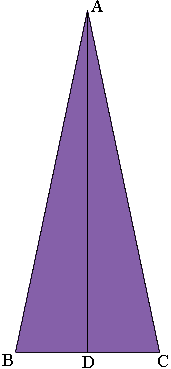SEARCH HOMEMath Central Quandaries & QueriesQuestion from Mariah, a student: An isosceles triangle has sides 10cm, 10 cm and the base 4cm. How do you find out the height of the triangle?Mariah,

Draw a diagram.D is the midpoint of BC. What does the symmetry tell you about the measure of the angle ADC? Can Pythagoras help?

PennyMath Central is supported by the University of Regina and The Pacific Institute for the Mathematical Sciences.